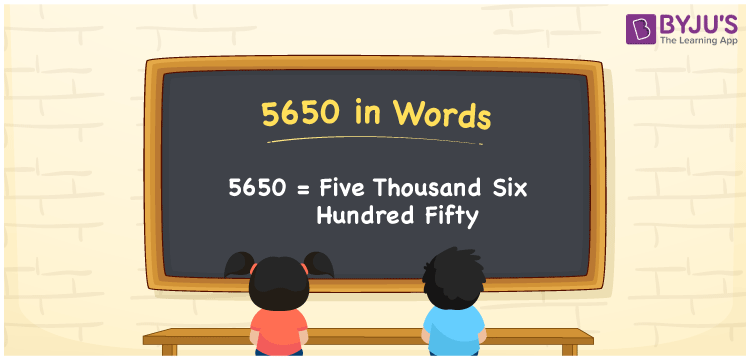# 5650 in Words

We can write 5650 in words as Five thousand six hundred fifty. If you bought a new broadband connection at Rs. 5650, then you can say, “I bought a new broadband connection for Five thousand six hundred fifty rupees”. Here, Five thousand six hundred fifty is the number name for 5650. In this article, you will get the conversion of the cardinal number to word form in an easily understandable method.

 5650 in words Five thousand six hundred fifty Five thousand six hundred fifty in Numbers 5650

## 5650 in English Words

Using the English alphabet, we generally write numbers in words. So, we can spell 5650 in English as “Five thousand six hundred fifty”.## How to Write 5650 in Words?

The number 5650 has four digits so we need to make a place value chart in such a way that all four digits of the number 5650 are defined with the place value.

 Thousands Hundreds Tens Ones 5 6 5 0

Here, ones = 0, tens = 5, hundreds = 6, and thousands = 5.

By expanding these digits, we get;

5 × Thousand + 6 × Hundred + 5 × Ten + 0 × One

= 5 × 1000 + 6 × 100 + 5 × 10 + 0 × 1

= 5000 + 600 + 50

= Five thousand + Six hundred + Fifty

= Five thousand six hundred fifty

Therefore, 5650 in words = Five thousand six hundred fifty.

As we know, 5650 is a natural number that precedes 5651 and succeeds 5649.

5650 in words – Five thousand six hundred fifty

Is 5650 an odd number? – No

Is 5650 an even number? – Yes

Is 5650 a prime number? – No

Is 5650 a composite number? – Yes

Is 5650 a perfect square number? – No

Is 5650 a perfect cube number? – No

## Frequently Asked Questions on 5650 in Words

Q1

### How do you write 5650 in words?

We can write the number 5650 in words as Five thousand six hundred fifty.
Q2

### How to write Rs. 5650 in words on a cheque?

On a cheque, we generally write Rs. 5650 in words as “Five thousand six hundred fifty rupees only”.
Q3

### What is the number name for 5650?

The number name for 5650 is Five thousand six hundred fifty.